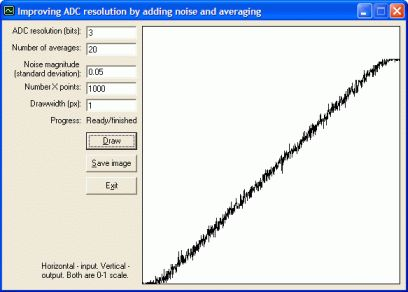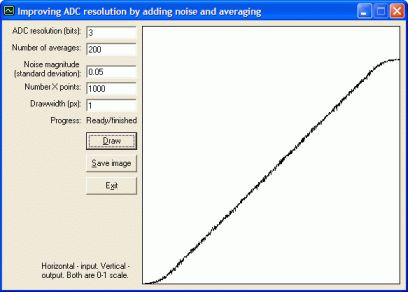Note: the following probably only applies to ADCs based on a successive approximation register (SAR). Other ADC topologies (e.g. Delta-Sigma) won't behave like this, or at least behave differently.

Suppose you have an analog signal and you're trying to digitise it using an analog-to-digital converter (ADC). If there is no noise present in the analog signal, a purely theoretical situation, then, no matter how many averages you take of the ADC output, the achievable resolution will remain the same. However, if a small amount of noise is added (or is present naturally), then taking averages of the ADC output can improve the resolution obtained. This trick is nothing new, but I couldn't find a simple demonstration of it, so wrote a wee program to show the effect. Program source and executable are available in this ZIP file (Visual Basic 6).

For the sake of argument, let's consider a 3-bit ADC. This will have 8 different possible output values. The screenshots of the program below show a graph of the averaged ADC output (if any averages are taken) versus the analog input. Both are in the range 0-1. When noise is added, it has a Gaussian distribution (generated with the Box-Muller transform) and its magnitude is defined by the standard deviation.

### 1. No averages, no noise

As expected, the output has 8 discrete steps.### 2. No averages, 0.02 noise

With 0.02 standard deviation of noise added, there are now noisy transitions around the boundaries between each output level.### 3. 20 averages, 0.02 noise

If we now take 20 averages of the noisy signal, note how a smoother slope has started to appear in between the main "steps" in the ADC output. More averages won't help to remove the remaining steps in the output.### 4. 20 averages, 0.05 noise

If yet more noise is added, the steps smooth out entirely into a linear slope.### 5. 200 averages, 0.05 noise

Increasing the averages helps to reduce the noise in the line.### Conclusion

Comparing the first and last images, you can clearly see how adding a small amount of noise and taking averages has hugely increased the achievable resolution of the ADC. Obviously, if too much noise is added, the benefit is reduced because you have to take more averages to get a smooth signal. It appears that the best amount of noise is a few LSBs of the original ADC output.